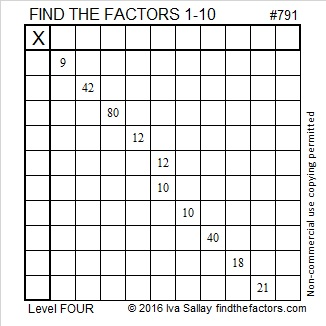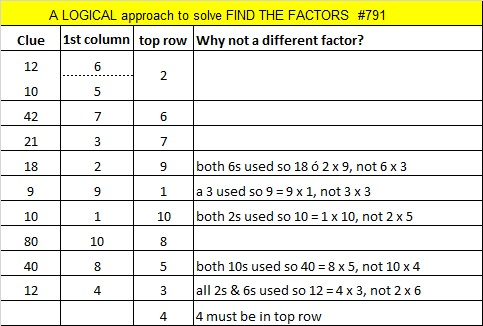# 791 and Level 4

To see if 791 is divisible by 7, you could try either one of these divisibility tricks:

• 791 is divisible by 7 because 79 – 2(1) = 77 which obviously is divisible by 7.
• 791 is divisible by 7 because 79 + 5(1) = 84 which most people know is 12 × 7.Print the puzzles or type the solution on this excel file: 10-factors-788-794

————————————–

• 791 is a composite number.
• Prime factorization: 791 = 7 x 113
• The exponents in the prime factorization are 1 and 1. Adding one to each and multiplying we get (1 + 1)(1 + 1) = 2 x 2 = 4. Therefore 791 has exactly 4 factors.
• Factors of 791: 1, 7, 113, 791
• Factor pairs: 791 = 1 x 791 or 7 x 113
• 791 has no square factors that allow its square root to be simplified. √791 ≈ 28.12472222.791 is the hypotenuse of Pythagorean triple 105-784-791 which is 15-112-113 times 7.

791 is also the sum of seven consecutive prime numbers:

• 101 + 103 + 107 + 109 + 113 + 127 + 131 = 791